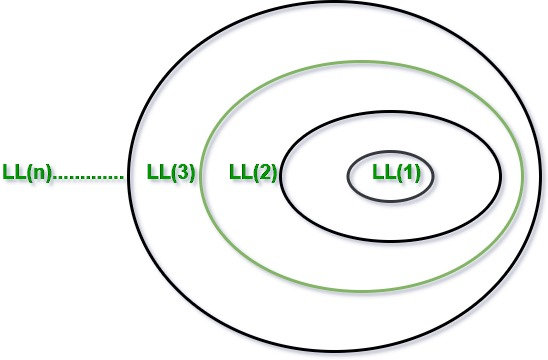Open In App
Related Articles
• Write an Interview Experience
• Compiler Design Tutorial

# Classification of Top Down Parsers

Parsing is classified into two categories, i.e. Top-Down Parsing, and Bottom-Up Parsing. Top-Down Parsing is based on Left Most Derivation whereas Bottom-Up Parsing is dependent on Reverse Right Most Derivation.

The process of constructing the parse tree which starts from the root and goes down to the leaf is Top-Down Parsing.

1. Top-Down Parsers constructs from the Grammar which is free from ambiguity and left recursion.
2. Top-Down Parsers use leftmost derivation to construct a parse tree.
3. It does not allow Grammar With Common Prefixes.

## Classification of Top-Down Parsing

1. With Backtracking: Brute Force Technique

2. Without Backtracking:

1. Recursive Descent Parsing
2. Predictive Parsing or Non-Recursive Parsing or LL(1) Parsing or Table Driver Parsing.

## Recursive Descent Parsing

1. Whenever a Non-terminal spends the first time then go with the first alternative and compare it with the given I/P String
2. If matching doesn’t occur then go with the second alternative and compare it with the given I/P String.
3. If matching is not found again then go with the alternative and so on.
4. Moreover, If matching occurs for at least one alternative, then the I/P string is parsed successfully.

Recursive Descent Parsing

`S(){     Choose any S production, S ->X1X2…..Xk;      for (i = 1 to k)      {          If ( Xi is a non-terminal)          Call procedure Xi();          else if ( Xi equals the current input, increment input)          Else /* error has occurred, backtrack and try another possibility */      }}`

Let’s understand it better with an example: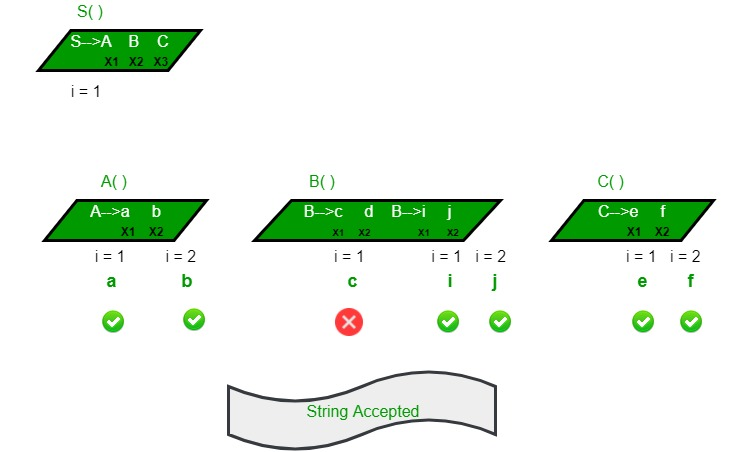A recursive descent parsing program consists of a set of procedures, one for each nonterminal. Execution begins with the procedure for the start symbol which halts if its procedure body scans the entire input string.

Non-Recursive Predictive Parsing: This type of parsing does not require backtracking. Predictive parsers can be constructed for LL(1) grammar, the first ‘L’ stands for scanning the input from left to right, the second ‘L’ stands for leftmost derivation, and ‘1’ for using one input symbol lookahead at each step to make parsing action decisions.

## LL(1) or Table Driver or Predictive Parser

1. In LL1, the first L stands for Left to Right, and the second L stands for Left-most Derivation. 1 stands for the number of Looks Ahead tokens used by the parser while parsing a sentence.
2. LL(1) parsing is constructed from the grammar which is free from left recursion, common prefix, and ambiguity.
3. LL(1) parser depends on 1 look ahead symbol to predict the production to expand the parse tree.
4. This parser is Non-Recursive

Construction of LL(1)predictive parsing table

For each production A -> α repeat the following steps:

• Add A -> α under M[A, b] for all b in FIRST(α)
• If FIRST(α) contains ε then add A -> α under M[A,c] for all c in FOLLOW(A).
• Size of parsing table = (No. of terminals + 1) * #variables

Example:

Consider the grammar
S → (L) | a
L → SL’
L’ → ε | SL’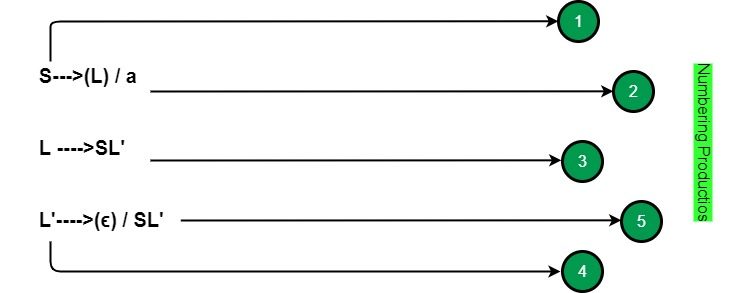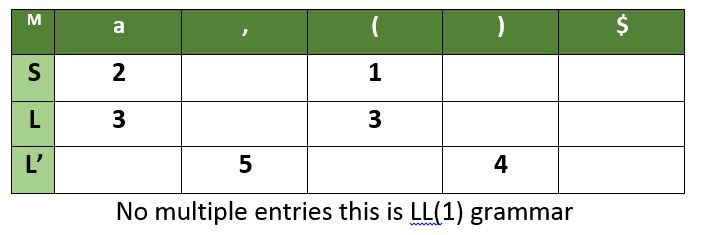For any grammar, if M has multiple entries then it is not LL(1) grammar.

Example:
S → iEtSS’/a
S’ →eS/ε
E → b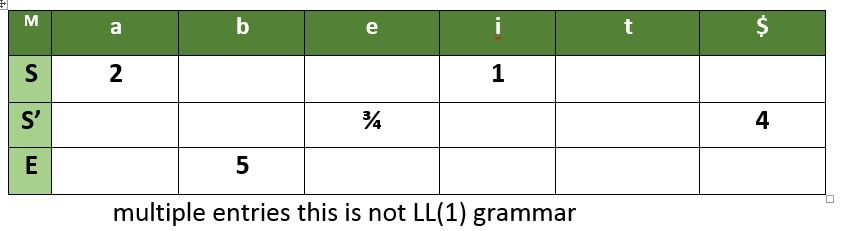Important Notes

If a grammar contains left factoring then it can not be LL(1).

`Eg - S -> aS | a      ---- both productions go in a`

If a grammar contains left recursion it can not be LL(1)

` Eg - S -> Sa | b       S -> Sa goes to FIRST(S) = b      S -> b goes to b, thus b has 2 entries hence not LL(1)`

If the grammar is ambiguous then it can not be LL(1).

Every regular grammar need not be LL(1) because regular grammar may contain left factoring, left recursion or ambiguity.